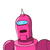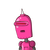# A rectangular sheet of paper 44cm×18cm is rolled along its length and a cylinder formed . Find Curved Surface Area of the cy

A rectangular sheet of paper 44cm×18cm is rolled along its length and a cylinder formed . Find Curved Surface Area
of the cylinder​

### 2 thoughts on “A rectangular sheet of paper 44cm×18cm is rolled along its length and a cylinder formed . Find Curved Surface Area<br /> of the cy”

1.## Step-by-step explanation:

Length of the rectangular sheet = Base circumference of the cylinder = 44cm

Breadth of the rectangular sheet = Height of the cylinder = 18cm

So, Curved Surface Area of the cylinder

$$= 2\pi rh$$

$$= 44 \times 18 \: {cm}^{2}$$

$$= 792 \: {cm}^{2}$$

Hence, Curved Surface Area of the cylinder is 792 cm^2.

2.Step-by-step explanation:

Let the height be 18cm

therefore, circumference of base 44cm